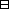## Group Theory and Linear Algebra

Last updated: 23 September 2014

## Lecture 18: Groups and group homomorphisms

A group is a set $G$ with a function $G×G ⟶ G (g1,g2) ⟼ g1g2$ such that

 (a) If ${g}_{1},{g}_{2},{g}_{3}\in G$ then ${g}_{1}\left({g}_{2}{g}_{3}\right)=\left({g}_{1}{g}_{2}\right){g}_{3},$ (b) There exists $1\in G$ such that if $g\in G$ then $1·g=g$ and $g·1=g\text{.}$ (c) If $g\in G$ then there exists ${g}^{-1}\in G$ such that $g·g-1=1and g-1·g=1.$

An abelian group is a set $A$ with a function $A×A ⟶ A (a1,a2) ⟼ a1+a2$ such that

 (a) If ${a}_{1},{a}_{2},{a}_{3}\in A$ then ${a}_{1}+\left({a}_{2}+{a}_{3}\right)=\left({a}_{1}+{a}_{2}\right)+{a}_{3},$ (b) There exists $0\in A$ such that if $a\in A$ then $0+a=a$ and $a+0=a\text{.}$ (c) If $a\in A$ then there exists $-a\in A$ such that $a+(-a)=0and (-a)+a=0,$ (d) If ${a}_{1},{a}_{2}\in A$ then ${a}_{1}+{a}_{2}={a}_{2}+{a}_{1}\text{.}$

Every abelian group is a group. $GL2(ℝ) = { 2×2 invertible matrices with entries in ℝ } = { (abcd) | a,b,c,d∈ℝ and ad-bc≠0 } .$ ${GL}_{2}\left(ℝ\right)$ is a group with product matrix multiplication. ${GL}_{2}\left(ℝ\right)$ is not an abelian group.

Let $G$ be a group. The order of $G$ is $\text{Card}\left(G\right),$ the number of elements in $G\text{.}$

The order of an element $g\in G$ is the smallest $k\in {ℤ}_{>0}$ such that ${g}^{k}=1\text{.}$ If there does not exist $k\in {ℤ}_{>0}$ such that ${g}^{k}=1$ then the order of $g\in G$ is $\infty \text{.}$

A subgroup of $G$ is a subset $H\subseteq G$ such that

 (a) If ${h}_{1},{h}_{2}\in H$ then ${h}_{1}{h}_{2}\in H\text{.}$ (b) $1\in H,$ (c) If $h\in H$ then ${h}^{-1}\in H\text{.}$

Group homomorphisms are for comparing groups.

Let $G$ and $K$ be groups. A group homomorphism from $K$ to $G$ is a function $f:K\to G$ such that if ${k}_{1},{k}_{2}\in K$ then $f\left({k}_{1}{k}_{2}\right)=f\left({k}_{1}\right)f\left({k}_{2}\right)\text{.}$

An isomorphism from $K$ to $G$ is a group homomorphism $f:K\to G$ such that there exists a group homomorphism $f-1:G\to K$ such that $f\circ f-1={\text{id}}_{G}$ and $f-1\circ f={\text{id}}_{K}\text{.}$

Let $f:K\to G$ be a group homomorphism. The kernel of $f$ is the set $ker f= {g∈G | f(g)=1}$ The image of $f$ is the set $im f= {f(g) | g∈G}.$

Let $f:K\to G$ be a group homomorphism. Then $f:K\to G$ is an isomorphism if and only if $f:K\to G$ is bijective.Proof.

$⇒$ Assume $f:K\to G$ is an isomorphism from $K$ to $G\text{.}$
To show: $f:K\to G$ is bijective.
Since $f$ is an isomorphism, there exists an inverse function to $f,$ $g:G\to K$ such that $g\circ f={\text{id}}_{G}$ and $f\circ g={\text{id}}_{K}\text{.}$
Thus, by theorem Theorem Let $f:K\to G$ be a function. An inverse function to $f$ exists if and only if $f$ is bijective. which is proved fully in Lecture notes, $f$ is bijective.
$⇐$ Assume $f:K\to G$ is a group homomorphism and $f:K\to G$ is bijective.
To show: $f:K\to G$ is an isomorphism.
To show:
 (a) There exists a function $g:G\to K$ such that $g∘f=idGand f∘g=idK.$ (b) $g:G\to K$ is a group homomorphism.
 (a) follows from Theorem. (b) To show: If ${x}_{1},{x}_{2}\in G$ then $g\left({x}_{1}{x}_{2}\right)=g\left({x}_{1}\right)g\left({x}_{2}\right)\text{.}$ Assume ${x}_{1},{x}_{2}\in G\text{.}$ To show: $g\left({x}_{1}{x}_{2}\right)=g\left({x}_{1}\right)g\left({x}_{2}\right)\text{.}$ Since $f$ is bijective, $f$ is injective, which means if ${k}_{1},{k}_{2}\in K$ and $f\left({k}_{1}\right)=f\left({k}_{2}\right)$ then ${k}_{1}={k}_{2}\text{.}$ To show: $f\left(g\left({x}_{1}{x}_{2}\right)\right)=f\left(g\left({x}_{1}\right)g\left({x}_{2}\right)\right)\text{.}$ $f(g(x1x2))= x1x2,$ since $f\circ g={\text{id}}_{G},$ and $f(g(x1)g(x2)) = f(g(x1)) f(g(x2)), since f is a homomorphism = x1·x2,since f∘g=idG.$ So $f\left(g\left({x}_{1}\right)g\left({x}_{2}\right)\right)=f\left(g\left({x}_{1}{x}_{2}\right)\right)\text{.}$ So $g\left({x}_{1}\right)g\left({x}_{2}\right)=g\left({x}_{1}{x}_{2}\right)\text{.}$ So $g$ is a homomorphism.

$\square$

## Notes and References

These are a typed copy of Lecture 18 from a series of handwritten lecture notes for the class Group Theory and Linear Algebra given on September 2, 2011.